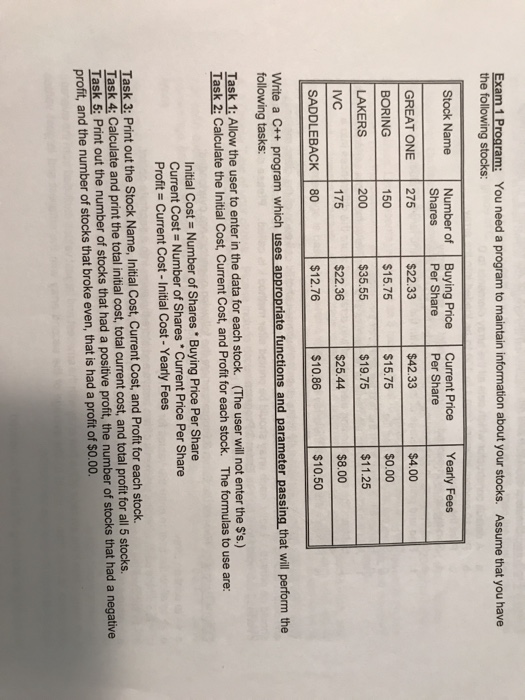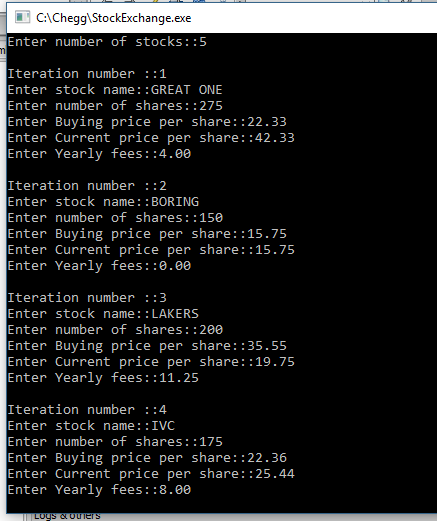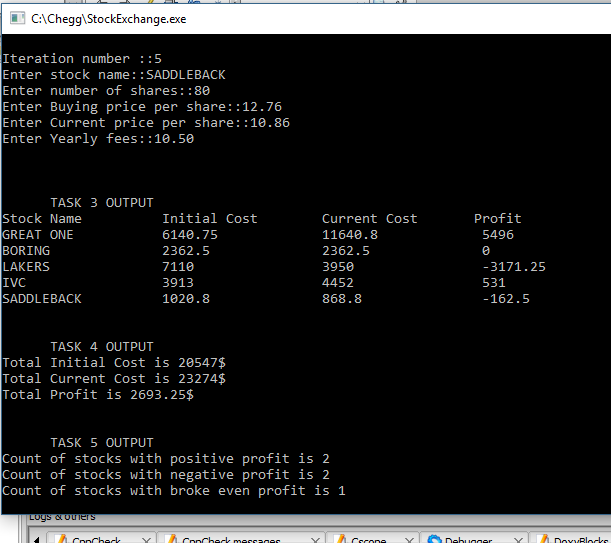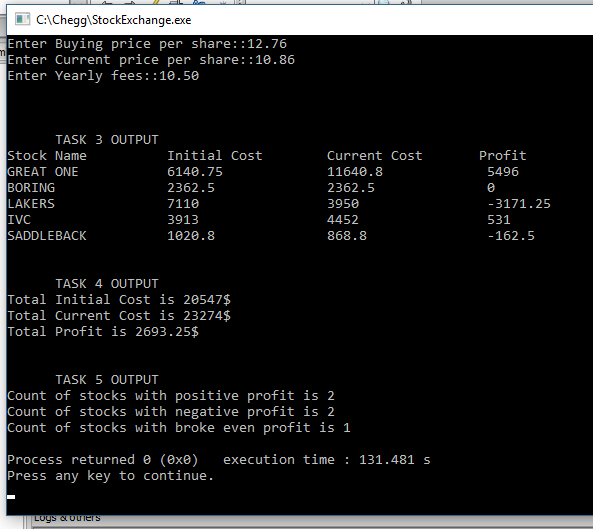# Answered Essay: Write a C++ program which uses appropriate functions and parameter passing.

Write a C++ program which uses appropriate functions and parameter passing.

Is it possible to write this program using only functions and parameter passing? Without StructExam 1 Program: You need a program to maintain information about your stocks. the following stocks: Assume that you have Stock Name Number of Buying Price Current Price Yearly Fees GREAT ONE 275 BORING LAKERS Per Share \$22.33 \$15.75 Shares Per Share \$42.33 \$15.75 \$19.75 \$25.44 \$10.86 \$4.00 150 200 175 \$0.00 |\$35.55 ー 151125 \$22.36 \$8.00 SADDLEBACK 80 \$12.76 \$10.50 Write a C++ program which uses appropriate functions and parameter passing that will perform the following tasks: Task 1: Allow the user to enter in the data for each stock. (The user will not enter the \$’s.) Task 2: Calculate the Initial Cost, Current Cost, and Profit for each stock. The formulas to use are: Initial Cost Number of Shares Buying Price Per Share Current Cost Number of Shares Current Price Per Share Profits Current Cost-Initial Cost-Yearly Fees Task 3: Print out the Stock Name, Initial Cost, Current Cost, and Profit for each stock. Task 4: Calculate and print the total initial cost, total current cost, and total profit for all 5 stocks. Task 5 Print out the number of stocks that had a positive profit, the number of stocks that had a negative profit, and the number of stocks that broke even, that is had a profit of \$0.00.

Explanation::

• Code is implemented in C++.
• Please read all the comments for better understanding of the code.
• Program is implemented in CodeBlocks IDE.
• Every step is well commented.
• Functions are created and their names are chosen in such a way that it becomes easy to identify it’s context.
• All variables declared have named according to context of the program.
• Output is provided below the code.

Code in c++::

#include<iostream>
#include<string>
#include <iomanip>
using namespace std;
void getInitialCost(double initialCost[],int numOfShares[],double buyingPrice[],int n);
void getCurrentCost(double currentCost[],int numOfShares[],double currentPrice[],int n);
void getProfit(double profit[],double currentCost[],double initialCost[],double yearlyFees[],int n);
void printData(string stockName[],double initialCost[],double currentCost[],double profit[],int n);
void totalValues(double initialCost[],double currentCost[],double profit[],int n);
void countValues(double profit[],int n);
int main(){
/*
* We need to use five arrays of size n
* which is taken as input as number of stocks.
*/
/*n is number of stocks*/
int n;
cout<<“Enter number of stocks::”;
cin>>n;

/*
* Now we declare string array named stockName
* of size n to stores names of the stock
*/
string stockName[n];

/*
* Now we declare integer array named numOfShares[]
* of size n to store number of shares.
*/
int numOfShares[n];

/*
* Now we declare double type array named buyingPrice[]
* of size n to store buying price per share.
*/

/*
* Now we declare double type array named currentPrice[]
* of size n to store current price per share.
*/
double currentPrice[n];

/*
* Now we declare double type array named yearlyFees[]
* of size n to store yearly fees.
*/
double yearlyFees[n];

/*
* ============== TASK 1 ENTERING DATA INTO ABOVE ARRAYS =============
* Using for loop we enter data for each stock in each iteration.
*/

for(int i=0;i<n;i++){
cout<<“nIteration number ::”<<(i+1)<<endl;
cout<<“Enter stock name::”;
/*
* To get the string with spaces I have used following getline function
*/
std::getline(std::cin >> std::ws, stockName[i]);

cout<<“Enter number of shares::”;
cin>>numOfShares[i];

cout<<“Enter Buying price per share::”;

cout<<“Enter Current price per share::”;
cin>>currentPrice[i];

cout<<“Enter Yearly fees::”;
cin>>yearlyFees[i];
}
cout<<endl;

/*
* ================== TASK 2 =================
* I am declaring three arrays named initialCost[], currentCost[] and profit[]
* All are of size n and they are of type double.
* Their value are calculated using function.
*/
double initialCost[n];
double currentCost[n];
double profit[n];

/*Calling respective functions to initialize above three arrays.*/
getCurrentCost(currentCost,numOfShares,currentPrice,n);
getProfit(profit,currentCost,initialCost,yearlyFees,n);

/*
* To print I have created printData() function that prints the data in
* required format. Required array are passed as parameters.
*/
/*
* Calling now function printData();
*/
cout<<“nn TASK 3 OUTPUT “<<endl;
printData(stockName,initialCost,currentCost,profit,n);

/*
* For task 4 I have also created on function that calculates
* total values and print in same function.
* Function name is totalValues()
*/
cout<<“nn TASK 4 OUTPUT “<<endl;
totalValues(initialCost,currentCost,profit,n);

/*
* I have created another function to get count of positive, negative and broke even
* Function name is countValues()
*/
cout<<“nn TASK 5 OUTPUT “<<endl;
countValues(profit,n);

return 0;
}
void getInitialCost(double initialCost[],int numOfShares[],double buyingPrice[],int n){
/*
* Calculates initial cost for each stock.
*/
for(int i=0;i<n;i++){
}
}
void getCurrentCost(double currentCost[],int numOfShares[],double currentPrice[],int n){
/*
* Calculates current cost for each stock.
*/
for(int i=0;i<n;i++){
currentCost[i]=numOfShares[i]*currentPrice[i];
}
}
void getProfit(double profit[],double currentCost[],double initialCost[],double yearlyFees[],int n){
/*
* Calculates profit for each stock.
*/
for(int i=0;i<n;i++){
profit[i]=currentCost[i]-initialCost[i]-yearlyFees[i];
}
}
void printData(string stockName[],double initialCost[],double currentCost[],double profit[],int n){
/*
* It just print the stocks with name,initial cost, current cost and profit with
* format specifiers.
*/
cout<<“Stock Name Initial Cost Current Cost Profit”<<endl;
/*
* Following variables are used to set spacing
*/
const char separator = ‘ ‘;
const int nameWidth = 20;
const int numWidth = 20;
for(int i=0;i<n;i++){
cout << left << setw(nameWidth) << setfill(separator) << stockName[i];
cout << left << setw(numWidth) << setfill(separator) << initialCost[i];
cout << left << setw(numWidth) << setfill(separator) << currentCost[i];
cout << left << setw(numWidth) << setfill(separator) << profit[i]<<endl;
// cout<<set” “<<stockName[i]<<” “<<initialCost[i]<<” “<<currentCost[i]<<” “<<profit[i]<<endl;
}
}
void totalValues(double initialCost[],double currentCost[],double profit[],int n){
/*
* It just calculate required total values and print them
*/
double totalInitialCost=0,totalCurrentCost=0,totalProfit=0;
for(int i=0;i<n;i++){
totalInitialCost+=initialCost[i];
totalCurrentCost+=currentCost[i];
totalProfit+=profit[i];
}
cout<<“Total Initial Cost is “<<totalInitialCost<<“\$”<<endl;
cout<<“Total Current Cost is “<<totalCurrentCost<<“\$”<<endl;
cout<<“Total Profit is “<<totalProfit<<“\$”<<endl;
}
void countValues(double profit[],int n){
/*
* CALCULATES POSITIVE NEGATIVE AND BROKE EVEN AND PRINT THEM
*/
int positiveCount=0,negativeCount=0,brokeEven=0;
for(int i=0;i<n;i++){
if(profit[i]<0){
negativeCount++;
}else if(profit[i]>0){
positiveCount++;
}else{
brokeEven++;
}
}
cout<<“Count of stocks with positive profit is “<<positiveCount<<endl;
cout<<“Count of stocks with negative profit is “<<negativeCount<<endl;
cout<<“Count of stocks with broke even profit is “<<brokeEven<<endl;

}

Output::Calculate your paper price
Pages (550 words)
Approximate price: -

Help Me Write My Essay - Reasons:Best Online Essay Writing Service

We strive to give our customers the best online essay writing experience. We Make sure essays are submitted on time and all the instructions are followed.Our Writers are Experienced and Professional

Our essay writing service is founded on professional writers who are on stand by to help you any time.Free Revision Fo all Essays

Sometimes you may require our writers to add on a point to make your essay as customised as possible, we will give you unlimited times to do this. And we will do it for free.Timely Essay(s)

We understand the frustrations that comes with late essays and our writers are extra careful to not violate this term. Our support team is always engauging our writers to help you have your essay ahead of time.Customised Essays &100% Confidential

Our Online writing Service has zero torelance for plagiarised papers. We have plagiarism checking tool that generate plagiarism reports just to make sure you are satisfied.Our agents are ready to help you around the clock. Please feel free to reach out and enquire about anything.

Try it now!

## Calculate the price of your order

Total price:
\$0.00

How it works?

Follow these simple steps to get your paper doneFill in the order form and provide all details of your assignment.Proceed with the payment

Choose the payment system that suits you most.Receive the final file

Once your paper is ready, we will email it to you.

HOW OUR ONLINE ESSAY WRITING SERVICE WORKS

Let us write that nagging essay.## Submit Your Essay/Homework Instructions

By clicking on the "PLACE ORDER" button, tell us your requires. Be precise for an accurate customised essay. You may also upload any reading materials where applicable.Pick A & Writer

Our ordering form will provide you with a list of writers and their feedbacks. At step 2, its time select a writer. Our online agents are on stand by to help you just in case.Editing (OUR PART)

At this stage, our editor will go through your essay and make sure your writer did meet all the instructions.